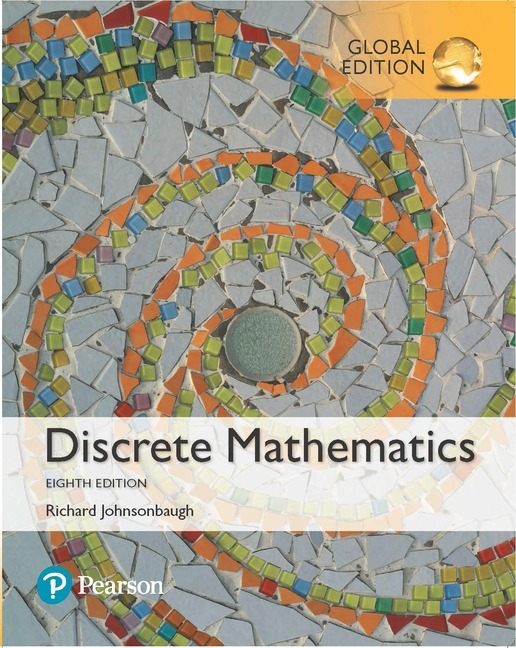•# Discrete Mathematics, Global Edition, 8th Edition

Regular price
RM 180.00
Sale price
RM 180.00
Regular price
RM 0.00

Richard Johnsonbaugh

For one- or two-term introductory courses in discrete mathematics.

Extend students’ mathematical maturity and ability to deal with abstraction

• Strong emphasis on reading and writing proofs — Illustrates most proofs of theorems with annotated figures to provide additional explanation and insight into the proofs.
• EXPANDED! More than 100 new exercises have been added to the first three chapters: Sets and Logic, Proofs, and Functions, Sequences, and Relations. There are now more than 1,750 worked examples and exercises in these chapters.
• Problem Solving Corners, a hallmark feature that helps students attack and solve problems and show them how to do proofs.
• Extensive discussion of algorithms, recursive algorithms, and the analysis of algorithms — The algorithms are written in a flexible form of pseudocode, which resembles currently popular languages such as C, C++, and Java.
• Extensive applications with an emphasis on computer science. Approximately 150 computer exercises are included throughout the book.
• Emphasis on the interplay among the various topics — For example, mathematical induction is closely tied to recursive algorithms; the Fibonacci sequence is used in the analysis of the Euclidean algorithm; many exercises throughout the book require mathematical induction; demonstrations of how to characterize the components of a graph by defining an equivalence relation on the set of vertices; and more.
• Figures and tables — Illustrate concepts, show how algorithms work, elucidate proofs, and motivate the material. Figure captions provide additional explanation and insight into figures accompanying proofs.
• Summaries of the mathematical and algorithmic notation used in the book on the inside covers.

1. Sets and Logic

1.1 Sets

1.2 Propositions

1.3 Conditional Propositions and Logical Equivalence

1.4 Arguments and Rules of Inference

1.5 Quantifiers

1.6 Nested Quantifiers

Problem-Solving Corner: Quantifiers

2. Proofs

2.1 Mathematical Systems, Direct Proofs, and Counterexamples

2.2 More Methods of Proof

Problem-Solving Corner: Proving Some Properties of Real Numbers

2.3 Resolution Proofs

2.4 Mathematical Induction

Problem-Solving Corner: Mathematical Induction

2.5 Strong Form of Induction and the Well-Ordering Property

3. Functions, Sequences, and Relations

3.1 Functions

Problem-Solving Corner: Functions

3.2 Sequences and Strings

3.3 Relations

3.4 Equivalence Relations

Problem-Solving Corner: Equivalence Relations

3.5 Matrices of Relations

3.6 Relational Databases

4. Algorithms

4.1 Introduction

4.2 Examples of Algorithms

4.3 Analysis of Algorithms

Problem-Solving Corner: Design and Analysis of an Algorithm

4.4 Recursive Algorithms

5. Introduction to Number Theory

5.1 Divisors

5.2 Representations of Integers and Integer Algorithms

5.3 The Euclidean Algorithm

Problem-Solving Corner: Making Postage

5.4 The RSA Public-Key Cryptosystem

6. Counting Methods and the Pigeonhole Principle

6.1 Basic Principles

Problem-Solving Corner: Counting

6.2 Permutations and Combinations

Problem-Solving Corner: Combinations

6.3 Generalized Permutations and Combinations

6.4 Algorithms for Generating Permutations and Combinations

6.5 Introduction to Discrete Probability

6.6 Discrete Probability Theory

6.7 Binomial Coefficients and Combinatorial Identities

6.8 The Pigeonhole Principle

7. Recurrence Relations

7.1 Introduction

7.2 Solving Recurrence Relations

Problem-Solving Corner: Recurrence Relations

7.3 Applications to the Analysis of Algorithms

8. Graph Theory

8.1 Introduction

8.2 Paths and Cycles

Problem-Solving Corner: Graphs

8.3 Hamiltonian Cycles and the Traveling Salesperson Problem

8.4 A Shortest-Path Algorithm

8.5 Representations of Graphs

8.6 Isomorphisms of Graphs

8.7 Planar Graphs

8.8 Instant Insanity

9. Trees

9.1 Introduction

9.2 Terminology and Characterizations of Trees

Problem-Solving Corner: Trees

9.3 Spanning Trees

9.4 Minimal Spanning Trees

9.5 Binary Trees

9.6 Tree Traversals

9.7 Decision Trees and the Minimum Time for Sorting

9.8 Isomorphisms of Trees

9.9 Game Trees

10. Network Models

10.1 Introduction

10.2 A Maximal Flow Algorithm

10.3 The Max Flow, Min Cut Theorem

10.4 Matching

Problem-Solving Corner: Matching

11. Boolean Algebras and Combinatorial Circuits

11.1 Combinatorial Circuits

11.2 Properties of Combinatorial Circuits

11.3 Boolean Algebras

Problem-Solving Corner: Boolean Algebras

11.4 Boolean Functions and Synthesis of Circuits

11.5 Applications

12. Automata, Grammars, and Languages

12.1 Sequential Circuits and Finite-State Machines

12.2 Finite-State Automata

12.3 Languages and Grammars

12.4 Nondeterministic Finite-State Automata

12.5 Relationships Between Languages and Automata

13. Computational Geometry

13.1 The Closest-Pair Problem

13.2 An Algorithm to Compute the Convex Hull

Appendix

A. Matrices B. Algebra Review C. Pseudocode

References

Hints and Solutions to Selected Exercises

Index